Electron. J. Diff. Eqns., Vol. 2007(2007), No. 93, pp. 1-47.

### Variational and topological methods for operator equations involving duality mappings on Orlicz-Sobolev spaces George Dinca, Pavel Matei

Abstract:
Let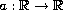be a strictly increasing odd continuous function with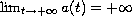and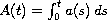,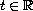, the N-function generated by a. Let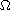be a bounded open subset of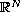,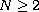,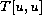a nonnegative quadratic form involving the only generalized derivatives of order m of the function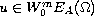and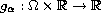,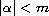, be Caratheodory functions.
We study the problem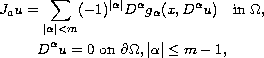where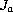is the duality mapping on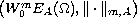, subordinated to the gauge function a (given by (1.5) and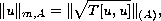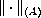being the Luxemburg norm on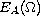.
By using the Leray-Schauder topological degree and the mountain pass theorem of Ambrosetti and Rabinowitz, the existence of nontrivial solutions is established. The results of this paper generalize the existence results for Dirichlet problems with p-Laplacian given in  and .

Submitted June 4, 2007. Published June 21, 2007.
Math Subject Classifications: 35B38, 35B45, 47J30, 47H11.
Key Words: A priori estimate; critical points; Orlicz-Sobolev spaces; Leray-Schauder topological degree; Duality mapping; Nemytskij operator; Mountain Pass Theorem.

Show me the PDF file (559 KB), TEX file, and other files for this article.George Dinca Faculty of Mathematics and Computer Science, University of Bucharest 14, Academiei Str., 010014 Bucharest, Romania email: dinca@fmi.unibuc.ro Pavel Matei Faculty of Mathematics and Computer Science, University of Bucharest 14, Academiei Str., 010014 Bucharest, Romania email: pavel.matei@gmail.com